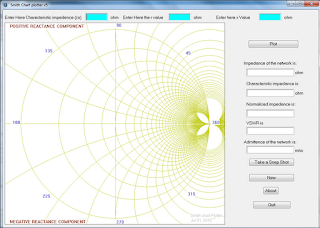## Sunday, July 3, 2011

### Smith chart plotter

This was my first ever major project. So this is going to be first blog pot. So what actually does is, plots the network characteristics on a smith chart. What is Smith chart you ask, it is a graphical tool that RF engineers use to evaluate the characteristics of a network more. For more info, head onto wikipedia  http://en.wikipedia.org/wiki/Smith_chart.

So why did I do it? Well at the time, I was UG engineering student and had just finished with TLWG (Transmission line and wave guides) and at about the same time, there was an EFY article explaining about the software that plotted Smith chart. It was based on the BGI libaray that came with the turbo C and I wanted to do something that was windows based and had a modern look and feel.

The language, environment that I choose to develop with was just BASIC(JB). which is a flavor of basic language and free version of commercial software called liberty BASIC. They are written in small talk. They are/were windows only software that is why I abandoned the platform.

So let us get on with the implementation of the software. The first few lines in the code  set up the GUI interface for us which is marked by "windows creation" section . The labels that you see within square brackets are actually event handlers. The flow jumps to these labels whenever event with which they are associated occurs. For example, you will find a label called new ([new]) which is the event handler for button named new. Each label ends with "wait" which reactivates the event handling loop. All the action happens when you click on the "plot" button. The event handler for this button first reads the input from the text boxes and then looks if they are valid data. If it sees that user has entered a valid data then it proceeds with the calculations. First it normalizes the impedance and then calculates the voltage standing wave ratio (VSWR).  With all this data, the next step is to plot the smith chart.

Before we get into the plotting logic, let us have a look at the drawing system used by JB. JB uses what is called as turtle graphics to draw stuffs. Conceptually, turtle (a turtle shaped object was used in some toy to draw pictures) has a pen and you can ask the turtle to move from (x1, y1) to (x2, y2) with pen up or pen down. If pen is down, it joins (x1, y1) with (x2, y2) else nothing appears. Additionally, this turtle also knows methods to draw graphics primitives such as circles, triangles etc.

First the program draws r-circles with predetermined radii. Then imaginary circles of the Smith chart are drawn just to give a smith chart kind of look. The subroutine that does this is "drawGraph". It also labels various parts in the graph. This sub routine calculates both radius and 'x' position for the center of the circle to be drawn. These calculations are made using the posx=(rl/(rl+1));  posR = (1.0/(rl+1)); where posx is the variable that holds the position for circle along x axis and posR is the radius of the circle and rl is the variable which is incremented within a for loop to get the concentric circles.  Similarly, the imaginary circles are drawn using the equation posix= = (1/rl) ; posR = (1/rl).

Once these circles are in place, the required circles that represent the network are drawn. First, the network impedance is normalized, then the VSWR is calculated  the equations used for calculations are those similar to the equations that appear in the wiki entry. All the above said calculations are scaled internally to suit the drawing area. Along with drawing the circle, the program also displays parameters such as normalized impedance, admittance of the network and VSWR.

You can download the software from here. I'll soon find and upload the source as well. I have uploaded the code to github.Smith chart plotter software